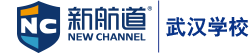400-009-9696

## SAT代数考点讲解

2017-12-18 16:55 来源：武汉编辑

ARITHMETIC
A. Whole numbers
1. Operations—addition, subtraction, multiplication, division
2. Prime and composite numbers
3. Factors and divisors
B. Fractions
1 Types—proper, improper, mixed numbers
2 Operations
C. Decimals
1 Operations
2 Conversions
a) Decimals to fractions
b) Fractions to decimals
3 Rounding and approximation
4 Powers of 10
a) Multiplication
b) Division
c) Scientific notation
D. Percent
1 Conversions
a) Percent to decimal
b) Decimal to percent
2 Percent problems
II ALGEBRA
A Signed numbers
1 absolute value
2 Inequality and order of signed numbers
3 Addition, subtraction, multiplication, division
4 Order of operations
5 Grouping symbols
6 Evaluating algebraic expressions and formulas
B. Properties of operations
1 Commutative properties
2 Associative properties
3 Distributive properties
4 Special properties of zero
5 Special properties of one
6 Additive and multiplicative inverses
C Operations with polynomials
1 Exponents and coefficients
2 Addition and subtraction
3 Multiplication
4 Division
D Equations in one variable
1 Methods of solution
2 Literal equationsE Inequalities in one variable
F Systems of equations and inequalities in two variables
G. verbal problems
1 number
2 Consecutive integer
3 Motion
4 Coin
5 Mixture
6 Age
7 Work
3 Variation—direct and inverse
H. Special products and factoring
1 Common monomial factors
2 Trinomials of the form ax2 + bx + c
3 Difference of two squares
4 Complete factoring I Algebraic fractions
1 Simplifying fractions
2 Multiplication
3 Division
4. Addition and subtraction
a) Same denominators
b) Different denominators
5 Complex fractions
6 Equations involving fractions
J Radicals and irrational numbers
1 Simplifying radicals
2 Addition and subtraction of radicals
3 Multiplication and division of radicals
4 Rationalizing denominators
5 Radical equations
6 Fractional exponents
K. Solution of quadratic equations
1 Factoring
2 Completing the square
3 Formula L. Graphing
1 Ordered pairs in the plane
2 Methods of graphing linear equations
a) Pairs in the solution set
b) Intercepts
c) Slope and slope-intercept method
3 Parallel and perpendicular lines
4 Graphing inequalities
5 Graphical solution of systems of equations
M Solution of simple cubic equations
1 Factor theorem
2 Remainder theorem
3 Synthetic division
4 Irrational and complex roots
5 Solving simple cubic equations

+86

+86

### 收不到验证码？

• 1.请检查是否输入正确的手机号码
• 2.检查手机是否停机
• 3.请使用其他账号登录
• 4.请联系官方客服

+86